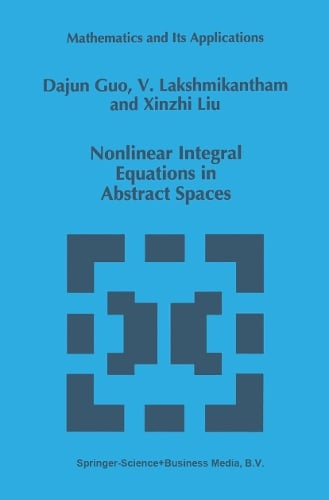•# Nonlinear Integral Equations in Abstract Spaces - Mathematics and Its Applications 373 (Paperback)

(author), (author), (author)
£69.99
Paperback 344 Pages / Published: 26/11/2013
• We can order this

Usually dispatched within 3 weeks

Many problems arising in the physical sciences, engineering, biology and ap plied mathematics lead to mathematical models described by nonlinear integral equations in abstract spaces. The theory of nonlinear integral equations in ab stract spaces is a fast growing field with important applications to a number of areas of analysis as well as other branches of science. This book is devoted to a comprehensive treatment of nonlinear integral equations in abstract spaces. It is the first book that is dedicated to a systematic development of this subject, and it includes the developments during recent years. Chapter 1 introduces some basic results in analysis, which will be used in later chapters. Chapter 2, which is a main portion of this book, deals with nonlin ear integral equations in Banach spaces, including equations of Fredholm type, of Volterra type and equations of Hammerstein type. Some applica equations tions to nonlinear differential equations in Banach spaces are given. We also discuss an integral equation modelling infectious disease as a typical applica tion. In Chapter 3, we investigate the first order and second order nonlinear integro-differential equations in Banach spaces including equations of Volterra type and equations of mixed type. Chapter 4 is devoted to nonlinear impulsive integral equations in Banach spaces and their applications to nonlinear impul sive differential equations in Banach spaces.

Publisher: Springer-Verlag New York Inc.
ISBN: 9781461285472
Number of pages: 344
Weight: 545 g
Dimensions: 235 x 155 x 19 mm
Edition: Softcover reprint of the original 1st ed. 199